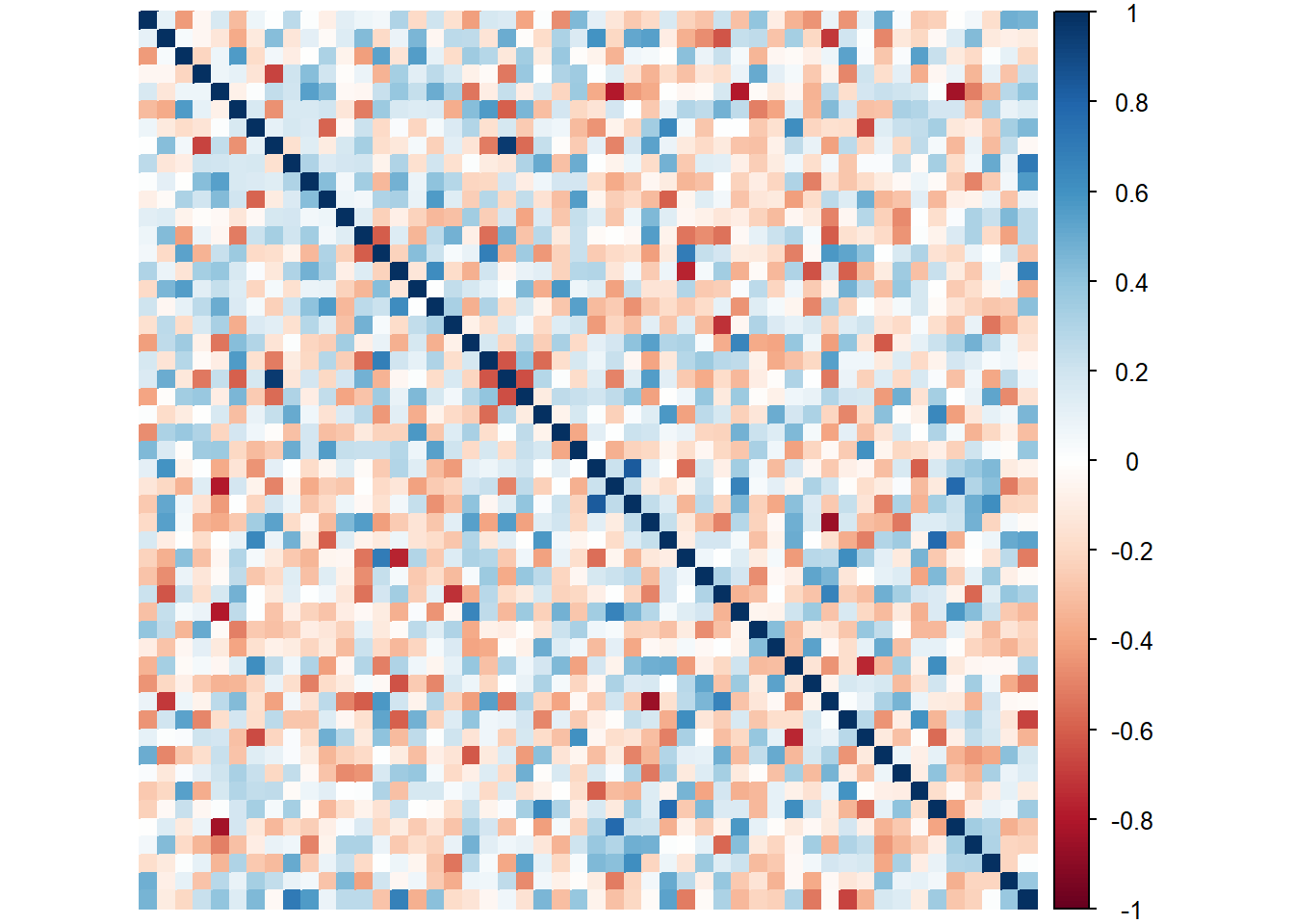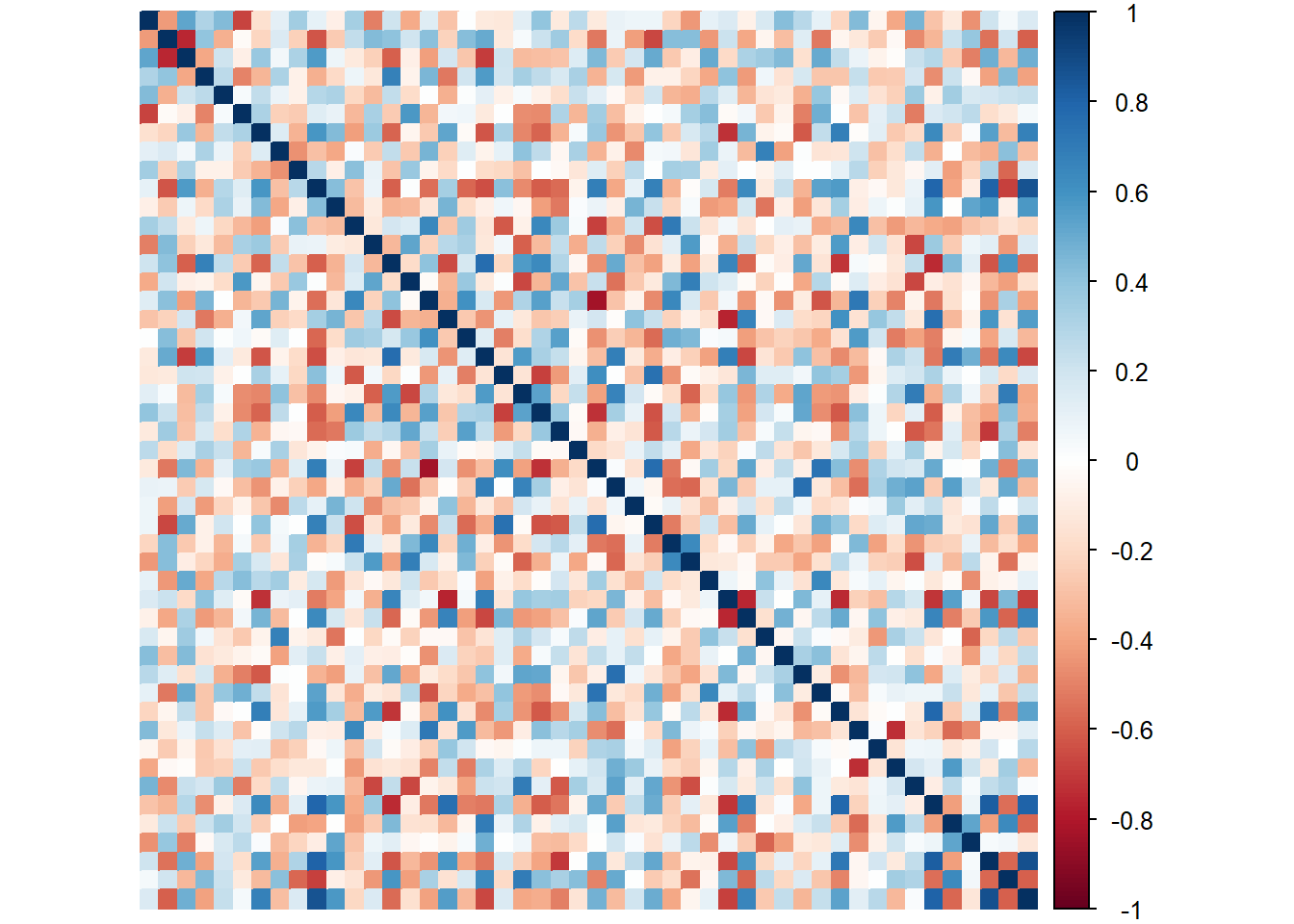## 9.3Correlation Plots

Like in other techniques, we will plot the heatmap of the 2 data tables we get the general feel of the data. We learned that the relationships among items (Judges) in each datasets is a mix of both positive and negative (leaning negative).

### 9.3.1 of Product Ratings

``````# Compute the covariance matrix
heat1 <- cor(sort)

# Plot it with corrplot
corrplot(heat1, method = "color",
#title = "Correlation Plot of Product Ratings",
tl.pos='n') ````````````#record
heatmap1 <- recordPlot()``````

### 9.3.2 of Vocab